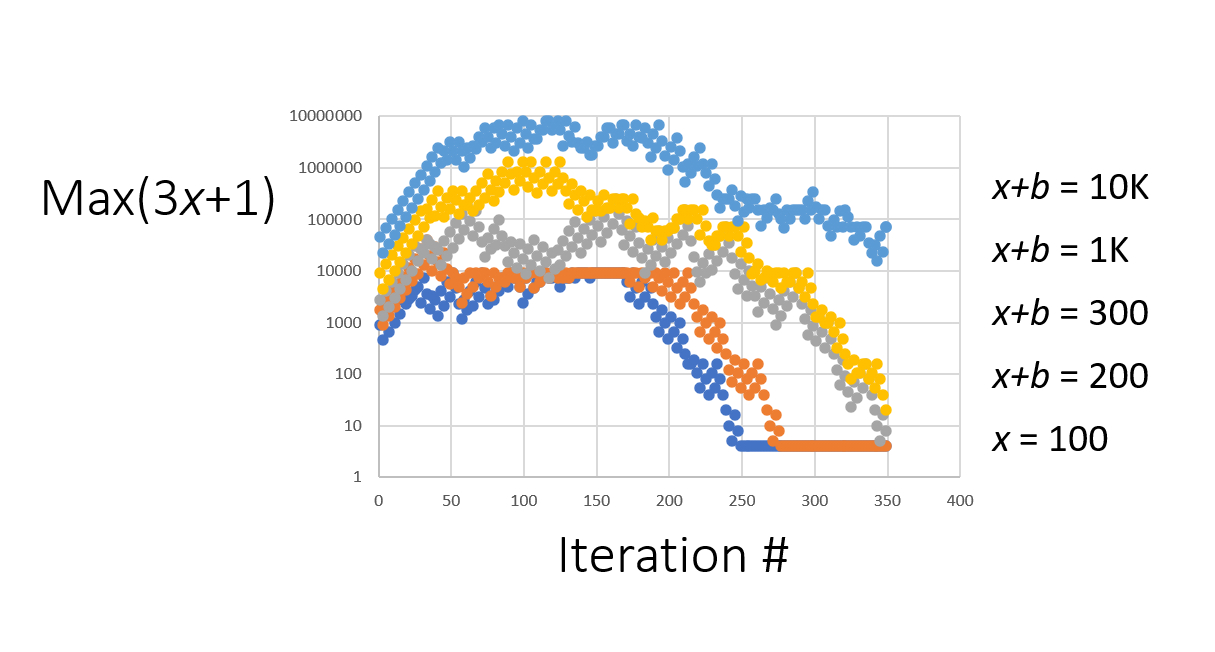# On The 3x+1 Problem

Sample number space of positive integer x’s between 1 and any arbitrary (positive) integer) n, and find Max(3x+1) for each iteration for all trials for all values of x between 1 and n.

.
For any arbitrary large b, there is always an equal or larger Max(3(x+b)+1) between 1 and (x+b) compared to that found between 1 and x.

Therefore, As (x+b) -> infinity, (3(x+b)+1) -> infinity.

COROLLARY
If one samples the same positive integer number space between 1 and any arbitrary n, one finds Max(Stopping I) for each iteration for all trials involving 1 to n.

For any arbitrary large b, there is always an equal or larger Max(Stopping I) between 1 and (x+b) compared to 1 and x.

This tells us that as (x+b) -> infinity, (Stopping I) -> infinity

Cf proof: For any positive values of x and y, (x+y) > x. Further, as y -> infinity, (x+y) -> infinity.

I hope this helps.

James Lyons-Weiler

Allison Park, PA

8/2/2021